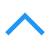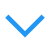# 深度学习网络层之 Batch Normalization

## Batch Normalization

S. Ioffe 和 C. Szegedy 在2015年《Batch Normalization: Accelerating Deep Network Training by Reducing Internal Covariate Shift》论文中提出此方法来减缓网络参数初始化的难处.

### Batch Norm原理

1）对特征的每个维度进行标准化，忽略白化中的去除相关性；
2）在每个mini-batch中计算均值和方差来替代整体训练集的计算。

batch normalization中即使不对每层的输入进行去相关，也能加速收敛。通俗的理解是在网络的每一层的输入都先做标准化预处理(公式中k为通道channel数),再向整体数据的均值方差方向转换.

$\hat x^{(k)}=\frac{x^{(k)}-E[x^{(k)}]}{\sqrt{\operatorname{Var[x^{(k)}]}}}$

Batch Normalizing Transform 转换式子:

\begin{align} \mu &= \frac{1}{m}\sum_{i=1}^m x_i &\text{//mini-batch mean} \\ \sigma^2 &= \frac{1}{m} \sum_{i=1}^m (x_i - \mu)^2 &\text{//mini-batch variance} \\ \hat{x_i} &= \frac{x_i - u}{\sqrt{\sigma^2 + \epsilon}} &\text{//normalize} \\ y_i &= \gamma \hat{x_i} + \beta &\text{//scale and shift} \end{align}

\begin{align} \mathcal{L}_{\bf w}(X,Y) =& \mathbb{E}_{{\bf x} \sim } \left[ \sum_{i=1}^N \left( y_i - {\bf w}^\top {\bf x}_i \right)^2 \right] \\ =& \mathbb{E}_{{\bf x} \sim } \left[ \sum_{i=1}^N y_i^2 - 2 y_i {\bf w}^\top {\bf x}_i + ( {\bf w}^\top {\bf x}_i )^2 \right] \\ =& \mathbb{E}_{{\bf x} \sim } \left[ \sum_{i=1}^N y_i^2 - 2 y_i (w_1 x_1 + w_2 x_2 ) + ( w_1 x_1 + w_2 x_2 )^2 \right] \\ =& \sum_{i=1}^N y_i^2 - 2 y_i w_1 \mathbb{E}_{x_1 \sim } \big[x_1 \big] - 2 y_i w_2 \mathbb{E}_{x_2 \sim } \big[ x_2 \big] \\ & + w_1^2 \mathbb{E}_{x_1 \sim } \big[ x_1^2 \big] + w_2^2 \mathbb{E}_{x_2 \sim } \big[ x_2^2 \big] + 2 w_1 w_2 \mathbb{E}_{ {\bf x} \sim } \big[ x_1 x_2 \big] \\ \end{align}

$\mathcal{L}_{\bf w}(X,Y) = \sum_{i=1}^N y_i^2 + w_1^2 \mathbb{E}_{x_1 \sim } \big[ x_1^2 \big] + w_2^2 \mathbb{E}_{x_2 \sim } \big[ x_2^2 \big] \\$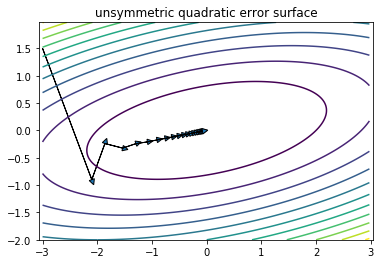1. 权重伸缩不变性

权重伸缩不变性（weight scale invariance）指的是，当权重 $\mathbf W$ 按照常量 $\lambda$ 进行伸缩时 $\mathbf W'=\lambda \mathbf W$，得到的规范化后的值保持不变, $Norm(\mathbf{W'}\mathbf{x})=Norm(\mathbf{W}\mathbf{x})$ . 从而

$\frac{\partial Norm(\mathbf{W'x})}{\partial \mathbf{W}} = \frac{\partial Norm(\mathbf{Wx})}{\partial \mathbf{W}}$

因此，权重的伸缩变化不会影响反向梯度的 Jacobian 矩阵，因此也就对反向传播没有影响，避免了反向传播时因为权重过大或过小导致的梯度消失或梯度爆炸问题，从而加速了神经网络的训练。因此权重伸缩不变性可以有效地提高反向传播的效率。

2. 数据伸缩不变性, 与权重不变性类似，输入数据发生伸缩变化时norm值不变可以有效地减少梯度弥散，简化对学习率的选择。每一层神经元的输出依赖于底下各层的计算结果。如果没有正则化，当下层输入发生伸缩变化时，经过层层传递，可能会导致数据发生剧烈的膨胀或者弥散，从而也导致了反向计算时的梯度爆炸或梯度弥散。

Batch Norm特征转换scale### 反向传播

\begin{aligned} \frac{\partial \ell}{\partial \widehat{x}_{i}} &=\frac{\partial \ell}{\partial y_{i}} \cdot \gamma \\ \frac{\partial \ell}{\partial \sigma_{\mathcal{B}}^{2}} &=\sum_{i=1}^{m} \frac{\partial \ell}{\partial \widehat{x}_{i}} \cdot\left(x_{i}-\mu_{\mathcal{B}}\right) \cdot \frac{-1}{2}\left(\sigma_{\mathcal{B}}^{2}+\epsilon\right)^{-3 / 2} \\ \frac{\partial \ell}{\partial \mu_{\mathcal{B}}} &=\left(\sum_{i=1}^{m} \frac{\partial \ell}{\partial \widehat{x}_{i}} \cdot \frac{-1}{\sqrt{\sigma_{\mathcal{B}}^{2}+\epsilon}}\right)+\frac{\partial \ell}{\partial \sigma_{\mathcal{B}}^{2}} \cdot \frac{\sum_{i=1}^{m}-2\left(x_{i}-\mu_{\mathcal{B}}\right)}{m} \\ \frac{\partial \ell}{\partial x_{i}} &=\frac{\partial \ell}{\partial \widehat{x}_{i}} \cdot \frac{1}{\sqrt{\sigma_{\mathcal{B}}^{2}+\epsilon}}+\frac{\partial \ell}{\partial \sigma_{\mathcal{B}}^{2}} \cdot \frac{2\left(x_{i}-\mu_{\mathcal{B}}\right)}{m}+\frac{\partial \ell}{\partial \mu_{\mathcal{B}}} \cdot \frac{1}{m} \\ \frac{\partial \ell}{\partial \gamma} &=\sum_{i=1}^{m} \frac{\partial \ell}{\partial y_{i}} \cdot \widehat{x}_{i} \\ \frac{\partial \ell}{\partial \beta} &=\sum_{i=1}^{m} \frac{\partial \ell}{\partial y_{i}} \end{aligned}

BN的前向与反向传播示意图: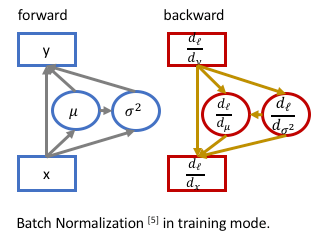\begin{align} \bar\mu &=\mathbb E[\mu_\mathcal B] \\ \bar\sigma^2 &={m\over m-1}\mathbb E[\sigma^2_\mathcal B] \end{align}

\begin{align} y&=γ({x-\bar\mu\over\sqrt{\bar\sigma^2+ϵ}})+ β \\ &={γ\over\sqrt{\bar\sigma^2+ϵ}}\cdot x+(β-{γ\bar\mu\over\sqrt{\bar\sigma^2+ϵ}}) \end{align}

### Batch Norm优点

• 减轻过拟合
• 改善梯度传播（权重不会过高或过低）
• 容许较高的学习率，能够提高训练速度。
• 减轻对初始化权重的强依赖，使得数据分布在激活函数的非饱和区域，一定程度上解决梯度消失问题。
• 作为一种正则化的方式，在某种程度上减少对dropout的使用。

### Batch Norm 应用

Batch Norm在卷积层的应用

1. 重新完全训练.如果想将BN添加到卷基层，通常要重新训练整个模型，大概花费一周时间。
2. finetune.只将BN添加到最后的几层全连接层，这样可以在训练好的VGG16模型上进行微调。采用ImageNet的全部或部分数据按batch计算均值和方差作为BN的初始$\beta,\gamma$参数。

### 与 Dropout 合作

Batch Norm的提出使得dropout的使用减少,但是Batch Norm不能完全取代dropout,保留较小的dropout率,如0.2可能效果更佳。

## Batch Norm 实现

Caffe框架的BatchNorm层参数设置示例:

layer {
name: "conv1/bn"
type: "BatchNorm"
bottom: "conv1"
top: "conv1"
param { lr_mult: 0 decay_mult: 0 } # mean
param { lr_mult: 0 decay_mult: 0 } # var
param { lr_mult: 0 decay_mult: 0 } # scale
batch_norm_param { use_global_stats: true } # 训练时设置为 false
}


Caffe框架中 BN 层全局均值和方差的实现：

\begin{align} \mu_{new} &= (\lambda \mu_{old} + \mu_B) / s \\ \sigma_{new}^2 &= \begin{cases} (\lambda \sigma_{old}^2 + \frac{m - 1}{m} \sigma_B^2)/s & m > 1 \\ (\lambda \sigma_{old}^2 + \sigma_B^2)/s & m = 1 \end{cases} \\ s &= \lambda s+1 \end{align}

caffe中的batch_norm_layer仅含均值方差,不包括gamma/beta,需要后边紧跟scale_layer,并使用bias来分别对应gamma、beta因子，用于自动学习缩放参数。

caffe实现的batch_norm_layer.cpp代码如下:

// scale初始化代码: 用三个blob记录BatchNorm层的三个数据
void BatchNormLayer<Dtype>::LayerSetUp(...) {
vector<int> sz;
sz.push_back(channels_);
this->blobs_.reset(new Blob<Dtype>(sz)); // mean
this->blobs_.reset(new Blob<Dtype>(sz)); // variance
// 在caffe实现中计算均值方差采用了滑动衰减方式, 用了scale_factor代替num_bn_samples(scale_factor初始为1, 以s=λs + 1递增).
sz = 1;
this->blobs_.reset(new Blob<Dtype>(sz)); // normalization factor (for moving average)
}

if (use_global_stats_) {
// use the stored mean/variance estimates.
const Dtype scale_factor = this->blobs_->cpu_data() == 0 ?
0 : 1 / this->blobs_->cpu_data();
caffe_cpu_scale(variance_.count(), scale_factor,
this->blobs_->cpu_data(), mean_.mutable_cpu_data());
caffe_cpu_scale(variance_.count(), scale_factor,
this->blobs_->cpu_data(), variance_.mutable_cpu_data());
} else {
// compute mean
caffe_cpu_gemv<Dtype>(CblasNoTrans, channels_ * num, spatial_dim,
1. / (num * spatial_dim), bottom_data,
spatial_sum_multiplier_.cpu_data(), 0.,
num_by_chans_.mutable_cpu_data());
caffe_cpu_gemv<Dtype>(CblasTrans, num, channels_, 1.,
num_by_chans_.cpu_data(), batch_sum_multiplier_.cpu_data(), 0.,
mean_.mutable_cpu_data());
}

// subtract mean
caffe_cpu_gemm<Dtype>(CblasNoTrans, CblasNoTrans, num, channels_, 1, 1,
batch_sum_multiplier_.cpu_data(), mean_.cpu_data(), 0.,
num_by_chans_.mutable_cpu_data());
caffe_cpu_gemm<Dtype>(CblasNoTrans, CblasNoTrans, channels_ * num,
spatial_dim, 1, -1, num_by_chans_.cpu_data(),
spatial_sum_multiplier_.cpu_data(), 1., top_data);

if (!use_global_stats_) {
// compute variance using var(X) = E((X-EX)^2)
caffe_powx(top->count(), top_data, Dtype(2),
temp_.mutable_cpu_data());  // (X-EX)^2
caffe_cpu_gemv<Dtype>(CblasNoTrans, channels_ * num, spatial_dim,
1. / (num * spatial_dim), temp_.cpu_data(),
spatial_sum_multiplier_.cpu_data(), 0.,
num_by_chans_.mutable_cpu_data());
caffe_cpu_gemv<Dtype>(CblasTrans, num, channels_, 1.,
num_by_chans_.cpu_data(), batch_sum_multiplier_.cpu_data(), 0.,
variance_.mutable_cpu_data());  // E((X_EX)^2)

// compute and save moving average
this->blobs_->mutable_cpu_data() *= moving_average_fraction_;
this->blobs_->mutable_cpu_data() += 1;
caffe_cpu_axpby(mean_.count(), Dtype(1), mean_.cpu_data(),
moving_average_fraction_, this->blobs_->mutable_cpu_data());
int m = bottom->count()/channels_;
Dtype bias_correction_factor = m > 1 ? Dtype(m)/(m-1) : 1;
caffe_cpu_axpby(variance_.count(), bias_correction_factor,
variance_.cpu_data(), moving_average_fraction_,
this->blobs_->mutable_cpu_data());
}

// normalize variance
caffe_powx(variance_.count(), variance_.cpu_data(), Dtype(0.5),
variance_.mutable_cpu_data());

// replicate variance to input size
caffe_cpu_gemm<Dtype>(CblasNoTrans, CblasNoTrans, num, channels_, 1, 1,
batch_sum_multiplier_.cpu_data(), variance_.cpu_data(), 0.,
num_by_chans_.mutable_cpu_data());
caffe_cpu_gemm<Dtype>(CblasNoTrans, CblasNoTrans, channels_ * num,
spatial_dim, 1, 1., num_by_chans_.cpu_data(),
spatial_sum_multiplier_.cpu_data(), 0., temp_.mutable_cpu_data());
caffe_div(temp_.count(), top_data, temp_.cpu_data(), top_data);

caffe_copy(x_norm_.count(), top_data,
x_norm_.mutable_cpu_data());


BN有合并式和分离式，各有优劣。

### BatchNorm层合并(Conv+BN+Scale+ReLU => Conv+ReLU)

bn layer: bn_mean, bn_variance, num_bn_samples 注意在caffe实现中计算均值方差采用了滑动衰减方式,用了scale_factor代替num_bn_samples(scale_factor初始为1,以s=λs+1递增).
scale layer: scale_weight, scale_bias 代表gamma,beta
BN层的batch的均值mu=bn_mean/num_bn_samples,方差var=bn_variance / num_bn_samples.
scale层设置新的仿射变换参数:

new_gamma = gamma / (np.power(var, 0.5) + 1e-5)
new_beta = beta - gamma * mu / (np.power(var, 0.5) + 1e-5)


Conv+BN+Scale=>Conv

conv layer: conv_weight, conv_bias

alpha = scale_weight / sqrt(bn_variance / num_bn_samples + eps)
conv_bias = conv_bias * alpha + (scale_bias - (bn_mean / num_bn_samples) * alpha)
for i in range(len(alpha)): conv_weight[i] = conv_weight[i] * alpha[i]


## Batch Norm 多卡同步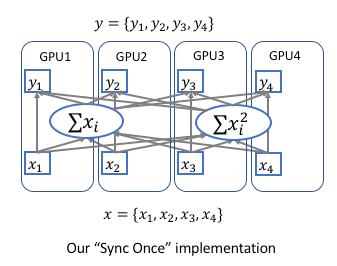\begin{align} \mu &= \frac{1}{m}\sum_{i=1}^m x_i \\ \sigma^2 &= \frac{1}{m} \sum_{i=1}^m (x_i - \mu)^2 = \frac{1}{m} \sum_{i=1}^m (x_i^2+\mu^2-2x_i\mu) = \frac{1}{m} \sum_{i=1}^m x_i^2 - \mu^2 \\ &= \frac{1}{m} \sum_{i=1}^m x_i^2 - (\frac{1}{m}\sum_{i=1}^m x_i)^2 \end{align}

posted @ 2017-09-22 19:55  康行天下  阅读(16574)  评论(11编辑  收藏  举报